1619269260

# Policy Gradient REINFORCE Algorithm with Baseline

Policy gradient methods are very popular reinforcement learning(RL) algorithms. They are very useful in that they can directly model the policy, and they work in both discrete and continuous space. In this article, we will:

1. have a short overview of the underlying math of policy gradient;
2. implement the policy gradient REINFORCE algorithm in Tensorflow to play CartPole;
3. compare Policy Gradient and Deep Q Network(DQN)

I assume readers have an understanding of reinforcement learning basics. As a refresher, you can take a quick look at the first section of my previous post A Structural Overview of Reinforcement Learning Algorithms.

I have also implemented Deep Q-net (DQN) in Tensorflow to play CartPole previously. Check it out here if you are interested. :)

## Buddha Community1619269260

## Policy Gradient REINFORCE Algorithm with Baseline

Policy gradient methods are very popular reinforcement learning(RL) algorithms. They are very useful in that they can directly model the policy, and they work in both discrete and continuous space. In this article, we will:

1. have a short overview of the underlying math of policy gradient;
2. implement the policy gradient REINFORCE algorithm in Tensorflow to play CartPole;
3. compare Policy Gradient and Deep Q Network(DQN)

I assume readers have an understanding of reinforcement learning basics. As a refresher, you can take a quick look at the first section of my previous post A Structural Overview of Reinforcement Learning Algorithms.

I have also implemented Deep Q-net (DQN) in Tensorflow to play CartPole previously. Check it out here if you are interested. :)1593766336

## Introduction to the Gradient Boosting Algorithm

The Boosting Algorithm is one of the most powerful learning ideas introduced in the last twenty years. Gradient Boosting is an supervised machine learning algorithm used for classification and regression problems. It is an ensemble technique which uses multiple weak learners to produce a strong model for regression and classification.

# Intuition

Gradient Boosting relies on the intuition that the best possible next model , when combined with the previous models, minimizes the overall prediction errors. The key idea is to set the target outcomes from the previous models to the next model in order to minimize the errors. This is another boosting algorithm(few others are Adaboost, XGBoost etc.).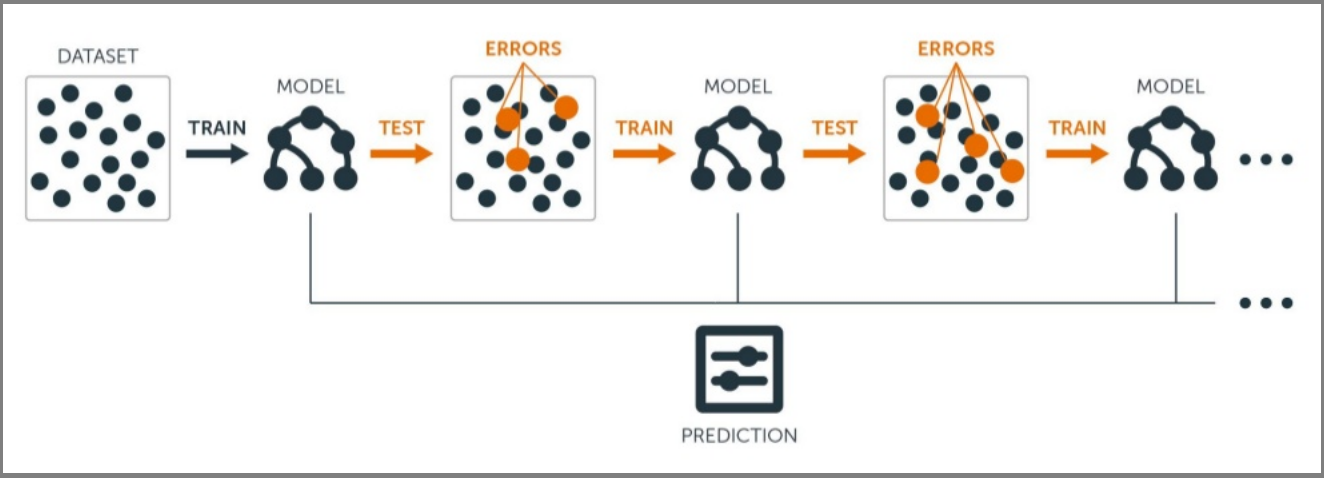1. A Loss Function to optimize.
2. A weak learner to make prediction(Generally Decision tree).
3. An additive model to add weak learners to minimize the loss function.

## 1. Loss Function

The loss function basically tells how my algorithm, models the data set.In simple terms it is difference between actual values and predicted values.

Regression Loss functions:

1. L1 loss or Mean Absolute Errors (MAE)
2. L2 Loss or Mean Square Error(MSE)

Binary Classification Loss Functions:

1. Binary Cross Entropy Loss
2. Hinge Loss

A gradient descent procedure is used to minimize the loss when adding trees.

## 2. Weak Learner

Weak learners are the models which is used sequentially to reduce the error generated from the previous models and to return a strong model on the end.

Decision trees are used as weak learner in gradient boosting algorithm.

In gradient boosting, decision trees are added one at a time (in sequence), and existing trees in the model are not changed.

# Understanding Gradient Boosting Step by Step :

This is our data set. Here Age, Sft., Location is independent variables and Price is dependent variable or Target variable.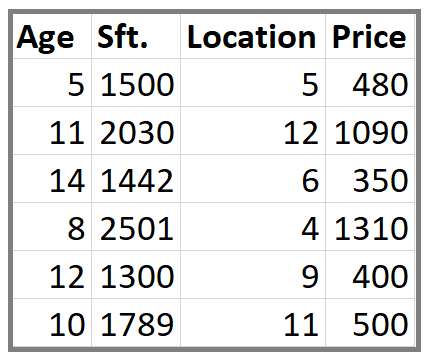Step 1: Calculate the average/mean of the target variable.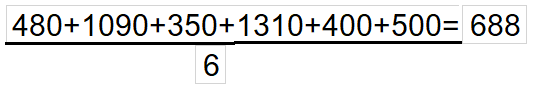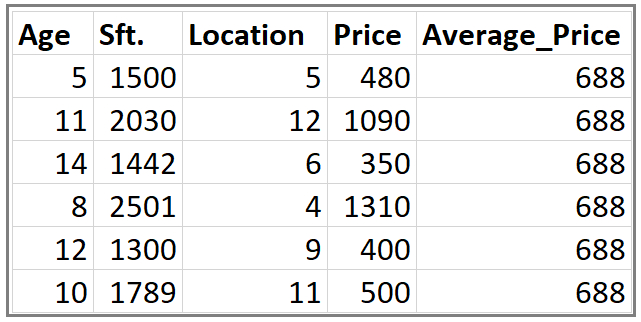Step 2: Calculate the residuals for each sample.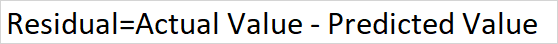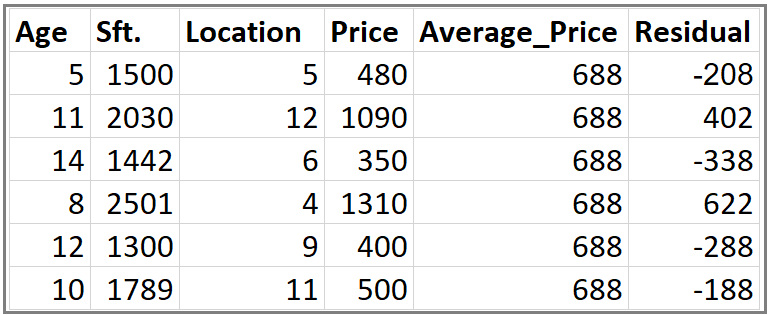**Step 3: **Construct a decision tree. We build a tree with the goal of predicting the Residuals.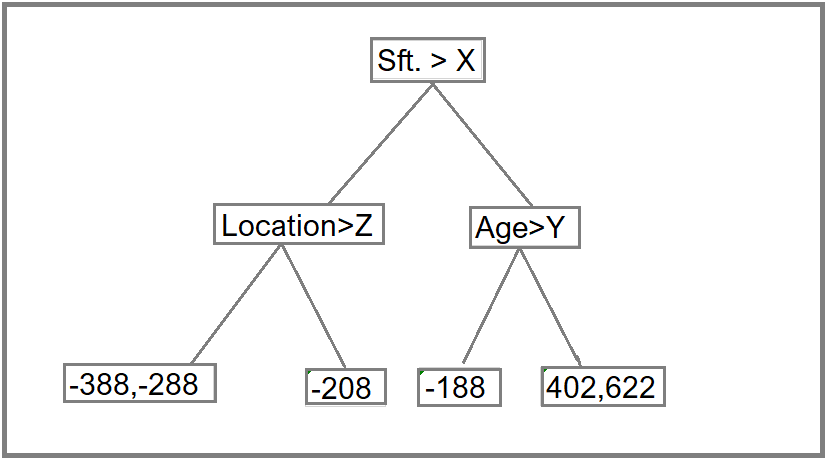In the event if there are more residuals then leaf nodes(here its 6 residuals),some residuals will end up inside the same leaf. When this happens, we compute their average and place that inside the leaf.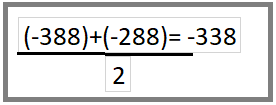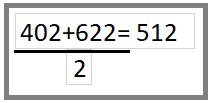After this tree become like this.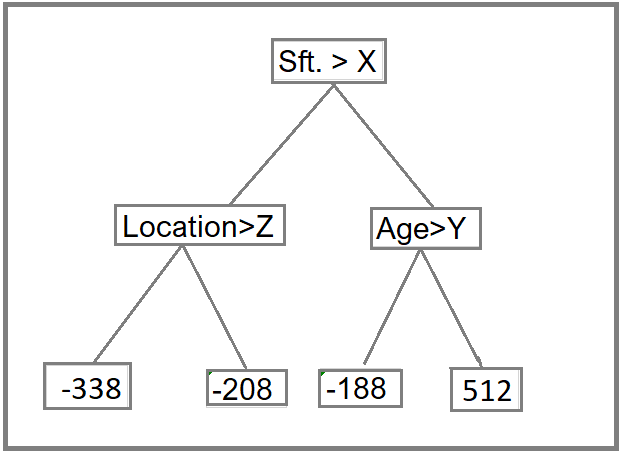Step 4: Predict the target label using all the trees within the ensemble.

Each sample passes through the decision nodes of the newly formed tree until it reaches a given lead. The residual in the said leaf is used to predict the house price.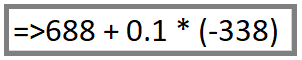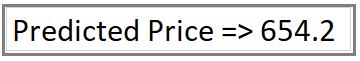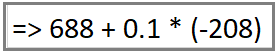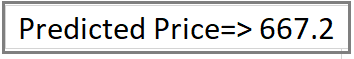Calculation above for Residual value (-338) and (-208) in Step 2

Same way we will calculate the Predicted Price for other values

Note: We have initially taken 0.1 as learning rate.

Step 5 : Compute the new residuals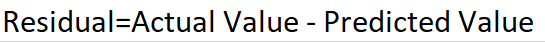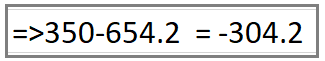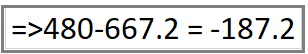When Price is 350 and 480 Respectively.1596428940

## Reducing Algorithmic Bias Through Accountability and Transparency

Despite being a mathematician’s dream word, algorithms — or sets of instructions that humans or, most commonly, computers execute — have cemented themselves as an integral part of our daily lives.

They are working behind the scenes when we search the web, read the news, discover new music or books, apply for health insurance, and search for a date. To put it simply, algorithms are a way to automate routine or information-heavy tasks.

However, some “routine” tasks have serious implications, such as determining credit scores, cultural or technical “fit” for a job, or the perceived level of criminal risk. While these algorithms are largely designed with society’s benefit in mind, algorithms are mathematical or logical models meant to reflect reality — which is often more nuanced than can be captured in a model.

For instance, some students aren’t eligible for loans because a lending model deems them too risky by virtue of their zip codes; which can result in an endless spiral of education and poverty challenges.

Algorithms can be incredibly helpful for society by improving human services, reducing errors, and identifying potential threats. However, algorithms are built by humans and thus reflect their creators’ imperfections and biases.

To ensure algorithms help society and do not discriminate, disparage, or perpetuate hate, we as a society, need to be more transparent and accountable in how our algorithms are designed and developed. Considering the importance of algorithms in our daily lives, here, a few examples of biased algorithms and how we can improve algorithm accountability.

# How computers learn biases

Much has been written on how humans’ cognitive biases influence everyday decisions. Humans use biases to reduce mental burden, often without cognitive awareness. For instance, we tend to think that the likelihood of an event is proportional to the ease with which we can recall an example of it happening. So if someone decides to continue smoking based on knowing a smoker who lived to be 100 despite significant evidence demonstrating the harms of smoking, that person is using what is called the availability bias.

Humans have trained computers to take over routine tasks for decades. Initially, these tasks were for very simple tasks, such as calculating a large set of numbers. As the computer and data science fields have expanded exponentially, computers are being asked to take on more nuanced problems through new tools (e.g., machine learning). Over time, researchers have found that algorithms often replicate and even amplify the prejudices of those who create them.

Since algorithms require humans to define exhaustive, step-by-step instructions, the inherent perspectives and assumptions can unintentionally build in bias. In addition to bias in development, algorithms can be biased if they are trained on incomplete or unrepresentative training data. Common facial recognition training datasets, for example, are 75% male and 80% white, which leads them to demonstrate both skin-type and gender biases, resulting in higher error rates and misclassification.

On a singular level, a biased algorithm can negatively impact a human life significantly (e.g., increasing the prison time based on race). When spread across an entire population, inequalities are magnified and have lasting effects on certain populations. Here are a few examples.

#policy #algorithms #technology #equality #social-justice #algorithms1593347004

## A greedy algorithm is a simple

The Greedy Method is an approach for solving certain types of optimization problems. The greedy algorithm chooses the optimum result at each stage. While this works the majority of the times, there are numerous examples where the greedy approach is not the correct approach. For example, let’s say that you’re taking the greedy algorithm approach to earning money at a certain point in your life. You graduate high school and have two options:

#computer-science #algorithms #developer #programming #greedy-algorithms #algorithms1596427800

## KMP — Pattern Matching Algorithm

Finding a certain piece of text inside a document represents an important feature nowadays. This is widely used in many practical things that we regularly do in our everyday lives, such as searching for something on Google or even plagiarism. In small texts, the algorithm used for pattern matching doesn’t require a certain complexity to behave well. However, big processes like searching the word ‘cake’ in a 300 pages book can take a lot of time if a naive algorithm is used.

# The naive algorithm

Before, talking about KMP, we should analyze the inefficient approach for finding a sequence of characters into a text. This algorithm slides over the text one by one to check for a match. The complexity provided by this solution is O (m * (n — m + 1)), where m is the length of the pattern and n the length of the text.

Find all the occurrences of string pat in string txt (naive algorithm).

``````#include <iostream>
#include <string>
#include <algorithm>
using namespace std;

string pat = "ABA"; // the pattern
string txt = "CABBCABABAB"; // the text in which we are searching

bool checkForPattern(int index, int patLength) {
int i;
// checks if characters from pat are different from those in txt
for(i = 0; i < patLength; i++) {
if(txt[index + i] != pat[i]) {
return false;
}
}
return true;
}

void findPattern() {
int patternLength = pat.size();
int textLength = txt.size();

for(int i = 0; i <= textLength - patternLength; i++) {
// check for every index if there is a match
if(checkForPattern(i,patternLength)) {
cout << "Pattern at index " << i << "\n";
}
}

}

int main()
{
findPattern();
return 0;
}
view raw
main6.cpp hosted with ❤ by GitHub
``````

# KMP approach

This algorithm is based on a degenerating property that uses the fact that our pattern has some sub-patterns appearing more than once. This approach is significantly improving our complexity to linear time. The idea is when we find a mismatch, we already know some of the characters in the next searching window. This way we save time by skip matching the characters that we already know will surely match. To know when to skip, we need to pre-process an auxiliary array prePos in our pattern. prePos will hold integer values that will tell us the count of characters to be jumped. This supporting array can be described as the longest proper prefix that is also a suffix.

#programming #data-science #coding #kmp-algorithm #algorithms #algorithms